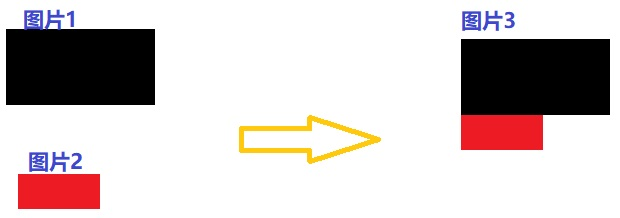2个回答

`````` def function(image1,image2):
h1,w1,c1 = image1.shape
h2,w2,c2 = image2.shape
if c1 != c2:
print("channels NOT match, cannot merge")
return
else:
if w1 > w2:
tmp = np.zeros([h2,w1-w2,c1])
image3 = np.hstack([image2,tmp])
image3 = np.vstack([image1,image3])
elif w1 == w2:
image3 = np.vstack([image1,image2])
else:
tmp = np.zeros([h1,w2-w1,c2])
image3 = np.hstack([image1,tmp])
image3 = np.vstack([image3,image2])
return image3
``````